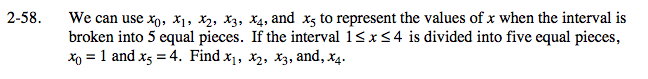### Home > PC > Chapter 2 > Lesson 2.2.2 > Problem2-58

2-58.Calculate the length of the interval: 4 − 1 = 3

Divide the length of the interval by the number of pieces: 3 ÷ 5 = 0.6 (length of each piece)

Start at 1 and add 0.6 to each interval.

x0 = 1
x1 = 1 + 0.6 = 1.6
x2 = 1.6 + 0.6 = 2.2
x3 = 2.2 + 0.6 = 2.8
x4 = 2.8 + 0.6 = 3.4